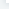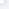# NumPy 位运算

NumPy 位运算NumPy "bitwise_" 开头的函数是位运算函数。NumPy 位运算包括以下几个函数：函数描述bitwise_and 对数组元素执行位与操作bitwise_or 对数组元素执行位或操作invert 按位取反left_shift 向左移动二进制表示的位right_shift 向右移动二进制表示的位注：也可以使用 "&"、 "~"、 "|" 和 "^" 等操作符进行计算。bitwise_andbitwise_and()函数对数组中整数的二进制形式执行位与运算。实例import numpy as npprint ('13 和 17 的二进制形式：')a,b = 13,17print (bin(a), bin(b))print ('\n')print ('13 和 17 的位与：')print (np.bitwise_and(13, 17))输出结果为：13 和 17 的二进制形式：0b1101 0b1000113 和 17 的位与：1以上实例可以用下表来说明：1101AND10001运算结果00001位与操作运算规律如下：ABAND111100010000bitwise_orbitwise_or()函数对数组中整数的二进制形式执行位与运算。实例import numpy as npa,b = 13,17print ('13 和 17 的二进制形式：')print (bin(a), bin(b))print ('13 和 17 的位或：')print (np.bitwise_or(13, 17))输出结果为：13 和 17 的二进制形式：0b1101 0b1000113 和 17 的位或：29以上实例可以用下表来说明：1101OR10001运算结果11101位或操作运算规律如下：ABOR111101011000invertinvert() 函数对数组中整数进行位取反运算，即 0 变成 1，1 变成 0。 对于有符号整数，取该二进制数的补码，然后 +1。二进制数，最高位为0表示正数，最高位为 1 表示负数。看看 ~1 的计算步骤：将1(这里叫：原码)转二进制 ＝ 00000001按位取反 ＝ 11111110发现符号位(即最高位)为1(表示负数)，将除符号位之外的其他数字取反 ＝ 10000001末位加1取其补码 ＝ 10000010转换回十进制 ＝ -2表达式二进制值（2 的补数）十进制值500000000 00000000 00000000 00000105~511111111 11111111 11111111 11111010-6实例import numpy as npprint ('13 的位反转，其中 ndarray 的 dtype 是 uint8：')print (np.invert(np.array(, dtype = np.uint8)))print ('\n')

# 比较 13 和 242 的二进制表示，我们发现了位的反转print ('13 的二进制表示：')print (np.binary_repr(13, width = 8))print ('\n')print ('242 的二进制表示：')print (np.binary_repr(242, width = 8))输出结果为：13 的位反转，其中 ndarray 的 dtype 是 uint8：13 的二进制表示：00001101242 的二进制表示：11110010left_shiftleft_shift() 函数将数组元素的二进制形式向左移动到指定位置，右侧附加相等数量的 0。实例import numpy as npprint ('将 10 左移两位：')print (np.left_shift(10,2))print ('\n')print ('10 的二进制表示：')print (np.binary_repr(10, width = 8))print ('\n')print ('40 的二进制表示：')print (np.binary_repr(40, width = 8))

# '00001010' 中的两位移动到了左边，并在右边添加了两个 0。输出结果为：将 10 左移两位：4010 的二进制表示：0000101040 的二进制表示：00101000right_shiftright_shift() 函数将数组元素的二进制形式向右移动到指定位置，左侧附加相等数量的 0。实例import numpy as npprint ('将 40 右移两位：')print (np.right_shift(40,2))print ('\n')print ('40 的二进制表示：')print (np.binary_repr(40, width = 8))print ('\n')print ('10 的二进制表示：')print (np.binary_repr(10, width = 8))

# '00001010' 中的两位移动到了右边，并在左边添加了两个 0。输出结果为：将 40 右移两位：1040 的二进制表示：0010100010 的二进制表示：00001010

NumPy 位运算

## 相关文章